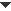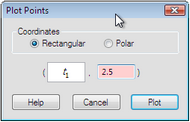﻿ Menus > Graph Menu > Plot Points/Plot as (x, y)/Plot as (r, θ)

# Plot Points/Plot as (x, y)/Plot as (r, θ)Plot as (x,y) / Plot as (r, θ)Plot PointsIf you don’t have two selected values, the command is Plot Points. This command displays a dialog box that allows you to plot one or more points on the marked coordinate system by entering the coordinates of each point to plot. In the dialog box: •Choose Rectangular to plot points using (x, y) values or choose Polar to plot them using (r, θ) values.•Click in either entry field and type numbers with decimal points, or type expressions such as 2+3 and π/4.•Click in either entry field and then click in the sketch on a value (or a Hot Text link to a value) to enter that value into the field.When you use a value from the sketch, the plotted point will move accordingly when the value changes. •Click Plot to plot the current values; the dialog box remains open to allow you to plot additional points.•After plotting your last point, click Done to close the dialog box.